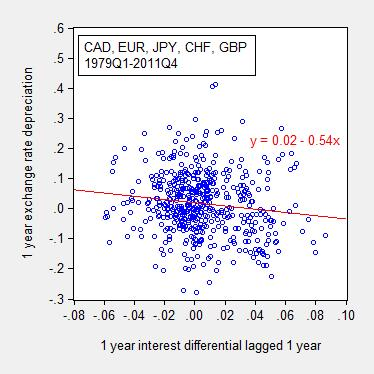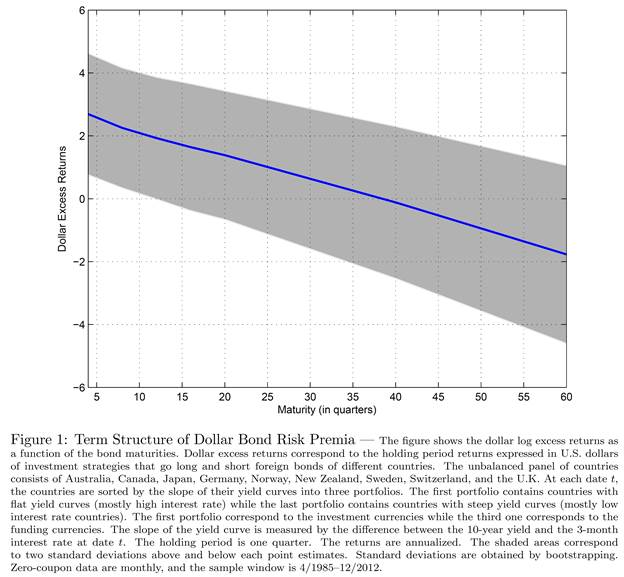# Forward Premia and the Carry Trade

I was at the NBER Summer Institute’s meeting of the International Finance and Macro group where (in addition to finally meeting Jim Hamilton) I had the opportunity to hear two papers on a topic near and dear to me — namely the relationship between the forward premium (the gap between the forward and spot rate, or equivalently in the absence of political risk, the interest differential) and the carry trade. (For discussion of related papers at last year’s IFM, see this post).

Recall, the basic issue is the negative correlation between interest differentials and the ex post depreciation over the corresponding time period. I plot the relationship at the 1 year horizon (for more regarding the data, see Chinn and Quayyum (2012), or this post).Figure 1: Pooled data for Canadian dollar, euro, Japanese yen, Swiss franc, and British pound against US dollar, 1979Q1-2011Q4. Note: “0.08” indicates 8%.

Notice that the zero arbitrage profits condition, under risk neutrality and rational expectations implies the slope of the red line should be upward sloping, with slope indistinguishable from unity. In contrast, as is well known, the slope at short maturities is typically negative, and statistically significantly so (see the survey in Chinn (2006)). In the graph above, the slope is -0.54, and statistically significantly different from zero (and from unity).

The first paper was by Tarek Hassan and Rui Mano, “Forward and spot exchange rates in a multi-currency world”. From the abstract:

We decompose violations of uncovered interest parity into a cross-currency, a between time-and-currency, and a cross-time component. We show that most of the systematic violations are in the cross-currency dimension. By contrast, we find no statistically reliable evidence that currency risk premia respond to deviations of forward premia from their time- and currency-specific mean. These results imply that the forward premium puzzle (FPP) and the carry-trade anomaly are separate phenomena that may require separate explanations. The carry trade is driven by static differences in interest rates across currencies, whereas the FPP appears to be driven primarily by cross-time variation in all currency risk premia against the US dollar. Models that feature two symmetric countries thus cannot explain either of the two phenomena. Once we make the appropriate econometric adjustments we also cannot reject the hypothesis that the elasticity of risk premia with respect to forward premia in all three dimensions is smaller than one. As a result, currency risk premia need not be correlated with expected changes in exchange rates.

Another way of putting this is that the carry trade is driven by differences in the constants in the Fama regression (along with what they term dynamic trade, based on variation in between-time-and-currency forward premia), while the forward premium trade relies upon the across-time covariation of exchange rate changes and the forward premia (along with the dynamic trade term).

The comments were various; one observer noted that these were unconditional correlations, while it’s known that some factors — including PPP deviations — are useful in predicting excess returns. My comment was that asymmetries were important — the expected UIP relationship holds when US interest rates are below foreign, and not when above (Bansal and Dahlquist).

Another interesting observation was that the decomposition, while useful, failed to illuminate a key question in the literature — where do these currency fixed effects come from. They’re apparently persistent, but don’t seem to be correlated with any particular observable macro variables.

The second paper was by Hanno Lustig, Andrea Stathopoulos, and Adrien Verdelhan, “The term structure of currency carry trade risk premium”. From the abstract:

We find that average returns to currency carry trades decrease significantly as the maturity of the foreign bonds increases, because investment currencies tend to have small local bond term premia. The downward term structure of carry trade risk premia is informative about the temporal nature of risks that investors face in currency markets. We show that long-maturity currency risk premia only depend on the domestic and foreign permanent components of the pricing kernels, since transitory currency risk is automatically hedged by interest rate risk for long-maturity bonds. Our findings imply that there is more cross-border sharing of permanent than transitory shocks.

One finding (based on a model which assumes importantly complete markets) is that excess returns on the dollar bonds appears as in Figure 1 from the paper.It is interesting that at a maturity of ten years, the premium is essentially zero. While these pertain to returns, and not yields to maturity, it is interesting that excess returns are zero at a horizon at which Chinn and Meredith (2004) find one cannot typically reject the unbiasedness hypothesis (uncovered interest parity combined with rational expectations). This pattern is shown in Figure 2.Figure 2: Beta coefficients from Fama regressions at different horizons. Source: Author’s calculations.

However, as pointed by my colleague Charles Engel, there are actually at least two interlinked puzzles regarding interest rates and exchange rates (paper here; post here). The first is the the tendency for exchange rates to appreciate when the interest differential is positive — which is addressed in the Lustig et al. paper. The second is the fact that the exchange rate tends to appreciate when the currency is strong (which pertains to the level of the exchange rate and subsequent change). That puzzle is not addressed in this paper.